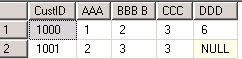### Convert Rows into Columns in SQL

I recently came across the following useful SQL query, Maybe you’ll find it useful.
In Sql, Pivoting can convert rows to columns. We will see these features in details in this post.
For example, we are considering a table named tblRowColumntoHere is SQL Query:
First, Create table called tblRowColumn
```CREATE TABLE [dbo].[tblRowColumn](
[custID] [int] NULL,
[Question] [varchar](50) NULL,
) ON [PRIMARY]
```

Then, insert some values..

```INSERT INTO tblRowColumn values(1000,    'AAA',    '1')
INSERT INTO tblRowColumn  values(1000,    'BBB B',    '2')
INSERT INTO tblRowColumn values(1000,    'CCC',    '3')
INSERT INTO tblRowColumn values(1001,    'AAA',    '2')
INSERT INTO tblRowColumn values(1001,    'BBB B',    '3')
INSERT INTO tblRowColumn values(1001,    'CCC',    '3')
INSERT INTO tblRowColumn values(1000,    'DDD',    '6')
```

here is pivot query to convert rows to columns..

```
Declare @A VARCHAR(1000)
Declare @B VARCHAR(1000)
set @A='SELECT CustID'
SET @B='('
SELECT @A=@A+',['+Question+'] as [' +Question+']',@B=@B+'['+Question+'],' FROM (SELECT DISTINCT QUESTION FROM tblRowColumn) cur
-- removing last ',' from both variables
SET @B=SUBSTRING(@B,1,LEN(@B)-1)
SET @A=@A+ + ' FROM (SELECT CustID, Answer, Question FROM tblRowColumn) s  PIVOT (max(answer) FOR Question IN ' +@B+')) p ORDER BY [CUSTID]; '
exec(@A);
```

Thanks to http://www.ashishblog.com/blog/how-to-convert-rows-into-columns-using-pivot-in-sql-serverquery/